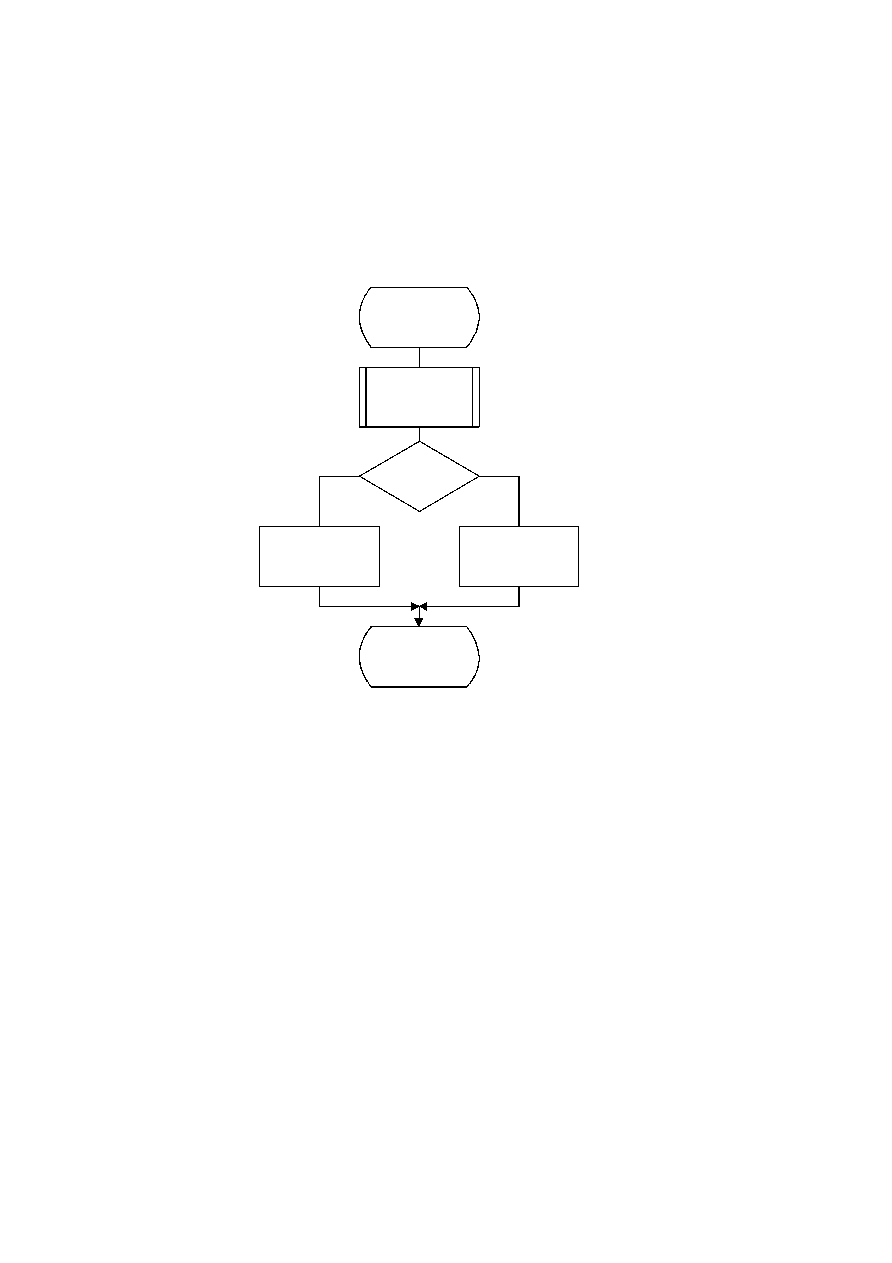ISO/IEC 10918-1 : 1993(E)
F.2.4.3.1.1
Decoding the sign
The sign is decoded by the procedure shown in Figure F.22.
The context-indices are defined for DC decoding in Table F.4 and AC decoding in Table F.5.
If SIGN
=
0, the sign of the coefficient is positive; if SIGN
=
1, the sign of the coefficient is negative.
TISO1540-93/d092
Decode_sign_of_V
SIGN = Decode(SS)
SIGN = 1
?
Yes
No
S = SN
S = SP
Done
Figure F.22 ­ Decoding the sign of V
Figure F.22 [D92] = 11 cm = 430 %
F.2.4.3.1.2
Decoding the magnitude category
The context-index S is set in Decode_sign_of_V and the context-index values X1 and X2 are defined for DC coding in
Table F.4 and for AC coding in Table F.5.
In Figure F.23, M is set to the upper bound for the magnitude and shifted left until the decoded decision is zero. It is then
shifted right by 1 to become the leading bit of the magnitude of Sz.
CCITT Rec. T.81 (1992 E)
115